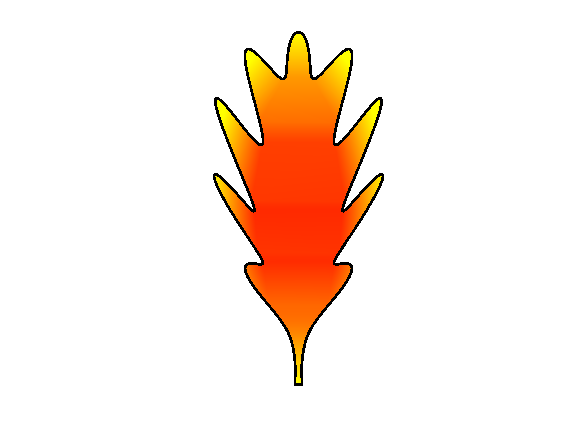# Oak LeafEric Ludlam on 29 Oct 2021
• 3
• 104
• 2
• 0
• 264
% Oak Leaf
% See https://blogs.scientificamerican.com/guest-blog/how-to-draw-with-math/
% and also "leaf pile" on File Exchange
t=0:0.01:pi;
x=1/100*(cos(t)).^9.*(cos(5*t)).^10+1/4*sin(2*t).*(1-1/2*(sin(10*t)).^2).*(1-(cos(t).*cos(3*t)).^8);
y=sin(t).*(1-1/5*(sin(10*t)).^2.*(1/2+(sin(2*t)).^2));
y=y-1/2;
z=0*t;
fill(x+rand*2,y+rand*2,hypot(x,y*.5),Linew=2);
axis equal off
colormap autumn
caxis([.1 .25])